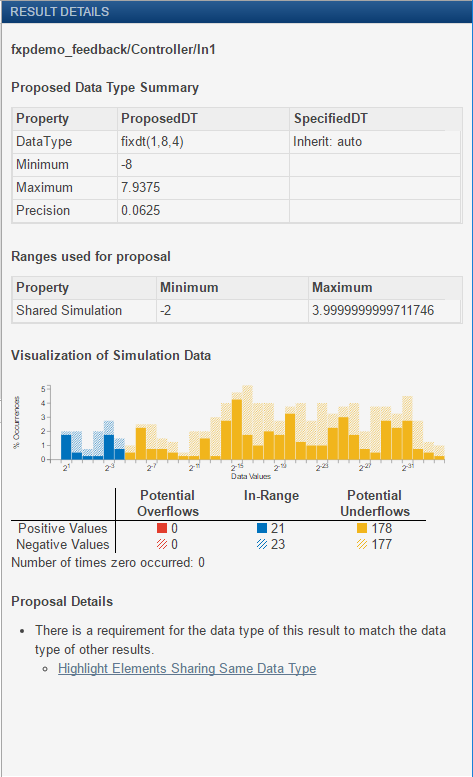Documentation

## View Simulation Results

The Fixed-Point Tool uses the Simulation Data Inspector tool plotting capabilities that enable you to plot logged signals for graphical analysis. Using the Simulation Data Inspector to inspect and compare data after converting your floating-point model to fixed point facilitates tracking numerical error propagation.

Use the Simulation Data Inspector to:

• Plot multiple signals in one or more axes

• Compare a signal in different runs

• Compare all logged signal data from different runs

• Export signal logging results to a MAT-file

• Specify tolerances for signal comparison

• Create a report of the current view and data in the Simulation Data Inspector

### Compare Runs

To compare runs:

1. In the Fixed-Point Tool, in the Verify section of the toolstrip, click.

2. If there are more than two runs, in the Compare Runs Selector dialog box, select the runs that you want to compare, and then click .

On the upper axes, the Simulation Data Inspector plots a signal in both selected runs. On the lower axes, the Simulation Data Inspector plots the difference between those runs.### Histogram Plot of Signal

To view the histogram plot of a signal:

1. In the Fixed-Point Tool, select the signal that you want to plot.

2. Click the Result Details tab.

The histogram plot helps you visualize the dynamic range of a signal. It provides information about the:

• Total number of samples (N).

• Maximum number of bits to prevent overflow.

• Number of times each bit has represented the data (as a percentage of the total number of samples).

• Number of times that exact zero occurred (without the effect of quantization). This number does not include the number of zeroes that occurred due to rounding.

You can use this information to estimate the word size required to represent the signal.## Related Topics

##### SupportGet trial now